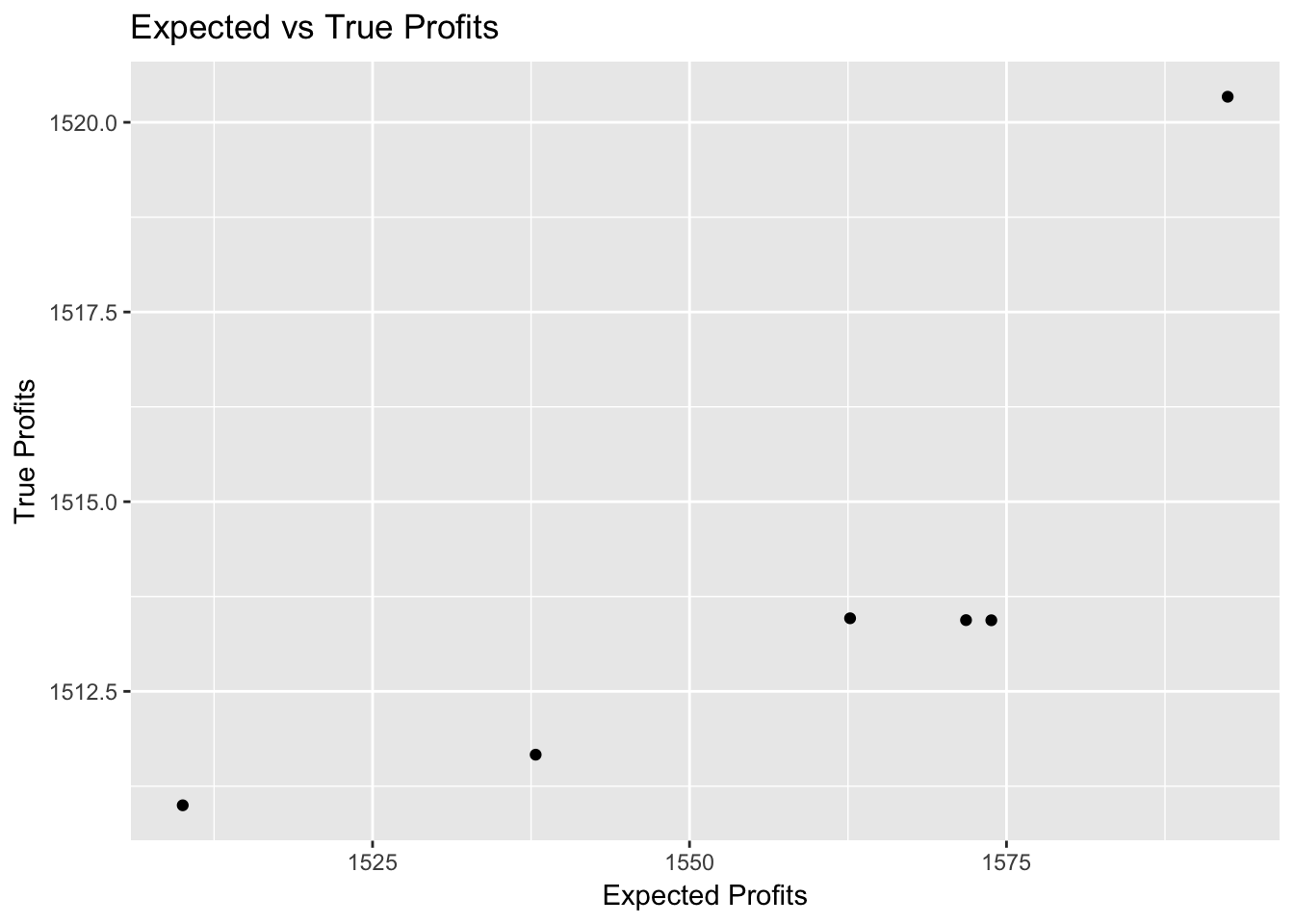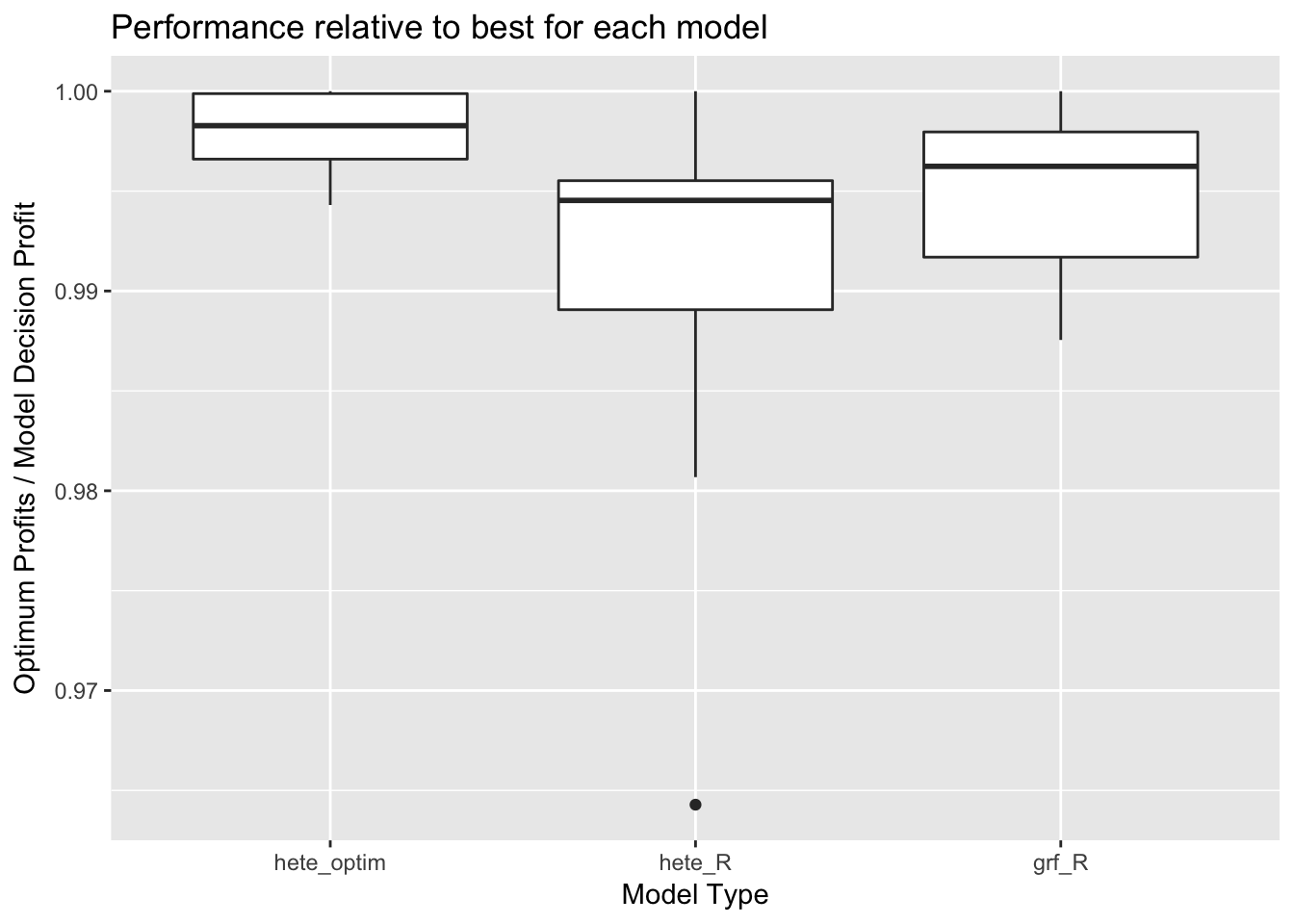## Monday, July 9, 2018

### Optimize for Expected Profit in Lift Models

hete is the wild west of analytics. no wrong answers yet - Bill Lattner

## Intro

Generally lift models use a Qini coefficient to measure the performance of a model. However this metric is generally an indirect measure of what the user wants to achieve: profit. In this post I’ll discuss a measure of profitability in lift models. A neural network can optimize this cost function directly. A comparison between this method compared with a causal tree implementation shows promising results.

## Current lift models

The goal of lift modeling (or Heterogeneous Treatment Effects ) is to assign an optimal action for a particular observation. This can be, among other things, targeted advertisement or price discrimination. There are several different formulations of lift models which include flip, causal trees, etc.
Essentially all try to estimate the interaction between covariates and the treatment. The most simple parameterized model is: : response
: covariates
: randomly assigned treatment variable. Assumed to be binary here.
${}_{}{}_{}{}_{}$
Here the treatment effect for a particular observation is ${}_{}{}_{}$. The key point here is that the treatment effect is now a function of  which means we can see which observations are more or less effected than others.
Alternatively one can estimate a machine learning model  and the treatment effect can be calculated $\stackrel{}{}\stackrel{}{}$.
However there are a few issues estimating this accurately which has spun several methods to accurately estimate it.
1. The heterogeneous treatment effect is probably very small. Indeed one may find that the treatment effect relative to main effect is so small it does not get split on in normal random forests / GBTs! This means we need a model to focus particularly on the treatment effect if we want the results to be useful.
2. We can have potentially hundreds of covariates available to include interactions with. We have no idea a priori which covariates are important so usually we have to expand parameter space significantly. Also, we don’t necessarily have the functional form available so we may take into account nonlinear transformations to the data.
3. We can’t compare the fitted treatment effect values to the ‘truth’. This is the biggest difference between lift models and normal prediction. In prediction settings we can set aside a test set and evaluate our predictions on known responses. Here we want to know the counterfactual; what would have happened if we gave an observation a different value, which lead us to the fundamental problem of causality.
So to sum up; we’re trying to estimate a very noisy signal, distributed among a large number of covariates, and we can’t check our estimated model directly.

## Metrics to use

Ok 3) might be a bit of a stretch because there are metrics people can use to assess the performance of their model. One is qini coefficient.
This is a measure that observations with similar treatment effects and groups them into the treatment they were given. If these groups are very different then that is a good measure the model is.
One particular drawback among qini coefficient is that it focuses only on the rank ordering of predicted values. This can be an adequate measure when you care about ranking but there are limitations. The most important being that it does not assign an obvious cutoff between those affected and those not. In the next section I will describe the problem in terms of cost / benefit and suggest a different metric.

## A new kind of metric

Suppose we have  options in the treatment and the goal is to assign the most profitable treatment for each observation. We have a model to assign an optimal treatment for observation : ${}_{}$. This takes the value 1 if  is best for observation  else it’s 0.The expected profit is:
$\underset{}{\overset{}{}}{}_{}{}_{}{}_{}$
The problem is that we cannot assign and observe a treatment to our training data. Instead we can only look at the values we have. Instead I will ‘simulate’ an experiment the following way:
$\frac{\underset{}{\overset{}{}}\underset{}{\overset{}{}}{}_{}{}_{}{}_{}}{\underset{}{\overset{}{}}\underset{}{\overset{}{}}{}_{}{}_{}}$
Basically this is saying if the optimal results equal the randomly assigned treatment then we include those in our hypothetical model. Since I am assuming the treatment is randomly assigned I think this would give a good indicator on the average increase for each observations under this new models decisions. In order to test this out I compared the expected gain with the actual gain of a simulated dataset. Below is the scatterplot and it appears to be a pretty good metric for the actual results.## Model this Metric Directly?

If we have a measure that can accurately measure what we want, can we use it as a loss function? This should lead to a better model since the model will optimize over the metric of interest.
We cannot optimize this directly b/c the indicator function is non-differentiable. Instead replacing I() function with a probability and use the custom function below to maximize. - Thanks to Matt Becker for this insight.
$\underset{}{\overset{}{}}\stackrel{}{{}_{}}{}_{}{}_{}$
This is essentialy answering the question: what is the probability that action  is best for observation ?

## Experiment

To test this new model (I’m calling it hete-optim) I simulated a similar dataset to that found in this paper. There are 200 variables with 15,000 training set and 3,000 test set. Of these there are 8 different scenarios using several nonlinear functions from the explanatory variables. One major difference is that I binarized the response. In addition I increased the random noise and decreased the relative treatment effect to get a more ‘realistic’
I’m comparing this model which my former colleague, Bill Lattner, Developed hete and to grf

# Results

The Metric I’m comparing is profits with true model / profits with fitted model. So if a model get’s a score of .75 then that means that it captures 75% of potential gain using a lift model. This is sort of a normalized regret score so we aggregate results among the 8 scenarios. Below is a boxplot of scores by model type.
## Using  as id variablesThis method performs best on 7 out of 8 datasets. On average hete_opim gets ~99.8% of the gains while hete gets ~98.9% and grf is 99.5%. This suggests that this method might be on to something.

# Conclusion

This post described a method to simulate profits in a real world lift model and the optimize it direclty. It shows promising results to existing techniques.